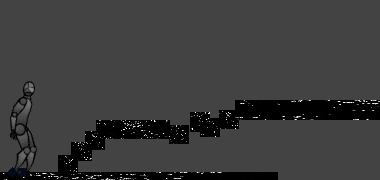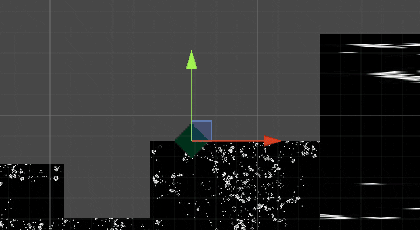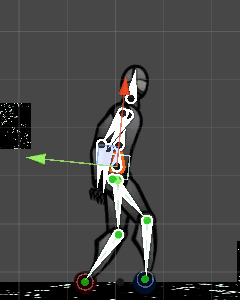Unity 2018.4.19f1Unknown LicenseUpdated 47 days agoCreated on September 2nd, 2020
Go to source

## Procedural animation showcase

Unity 2D humanoid procedural animation

## 1. Overview

This is the general explanation of the idea of simple 2D procedural leg animation for bipedal character. Project uses inverse kinematics (IK) to calculate position of the leg, which adapts to the terrain.Results

## 2. Idea

Legs of the rigged character are chain of the two bones, end of which (feet) are always trying to reach for the raycasted target after it reaches certain range. To prevent unnatural, direct movement from point to point of both legs, there is added logic, which controls and syncs leg and body movement depending on the environment and current placement of the feet.

## 3. Character rig

Bone rig for legs is simplified to make later calculations easier and each bone affects one sprite assigned to it. Top of the body could have no bones, but those will be important in further developement for arms and head animations.## 4. Raycasting desired position

There is an raycaster attached to the body which adjusts y coordinate of the desired position, represented here by the diamond icon, depending on the distance from the ground collider. It shows where leg will be placed if controller will be in certain range from current leg position.

``````using UnityEngine;

public class RaycastTarget : MonoBehaviour {
float desiredYPosition;
public Transform desiredTarget;

void Update() {
RaycastCollider();
desiredTarget.position = new Vector2(desiredTarget.position.x, desiredYPosition);
}

void RaycastCollider() {
RaycastHit2D hit = Physics2D.Raycast(new Vector2(desiredTarget.position.x, transform.position.y + 5), -Vector2.up, 12f);

if (hit.collider != null) {
desiredYPosition = hit.point.y;
}
else {
desiredYPosition = transform.position.y;
}
}
}
``````## 5. Inverse kinematics

It makes possible for us to calculate possible rotations of the bones, that will allow feet to reach desired point.Nearly all complicated calculations are diminished thanks to the simplified bone structure and reduction of the problem to the two dimensions. It allows us to use basic trigonometry instead of the iterating over matrix operations.We know lengths and positions of all the bones and all line segments, so it allows us to easily calculate what angle should be between red and blue bone, to reach designated point

## 6. Controlling and syncing the body

Body is controlled by another raycasted target, which moves around and rest of the body tries to be in middle of both leg points and followed target.

``````// moves body horizontally so it's in the middle of the three points
void MoveBody() {
Vector2 pos = transform.position;
float targetX = (((
rayTargetF.transform.position.x +
rayTargetB.transform.position.x +
legPoint.transform.position.x) / 3) -
transform.position.x) /
easeDiv;

pos.x += targetX;
transform.position = pos;
}
``````

Then we make check if both legs are in contact with the ground, if so, we can calculate distance from followed point, to leg targets. We are doing this to check which distance is greater, to determine which leg should be moved now.

``````// check if should make a step
void CheckRange() {
// calculate distance from followed point to leg targets
float distF = Vector2.Distance(legPoint.transform.position, frontLeg.transform.position);
float distB = Vector2.Distance(legPoint.transform.position, backLeg.transform.position);

// check if both legs are in contact with the ground
if (inContact == 2) {
// front leg is further away
if (distF > distB) {
float dist = Vector2.Distance(legPoint.transform.position, rayTargetB.transform.position);

if (dist > reachRange) {
rayTargetF.transform.position = legPoint.transform.position;
solverF.GetComponent<MoveTarget>().inContact = false;
inContact -= 1;
}
}
// back leg is further away
else {
float dist = Vector2.Distance(legPoint.transform.position, rayTargetF.transform.position);

if (dist > reachRange) {
rayTargetB.transform.position = legPoint.transform.position;
solverB.GetComponent<MoveTarget>().inContact = false;
inContact -= 1;
}
}
}
}
``````

To check if the character is grounded, we calculate how many legs are close enough to their target which is always on the ground.

``````void CheckContact() {
float targetDist = Vector2.Distance(transform.position, currentTarget.transform.position);

if (targetDist < reachRange * 0.05 && inContact == false) {
controller.GetComponent<BodyControl>().inContact += 1;
inContact = true;
}
}
``````After all those checks we can move our leg effector towards the desired position. It’s done using simple `Vector2.MoveTowards()` function combined with adjustable easing out function, to make movement more natural.

``````float targetX = currentTarget.position.x;
float targetY = currentTarget.position.y;

Vector2 targetPointFinal = new Vector2(targetX, targetY);

float xChange = (currentTarget.transform.position.x - transform.position.x) / easeDiv / 2;

// two movement functions
transform.position = Vector2.MoveTowards(transform.position, targetPointFinal, speed * Time.deltaTime);
transform.position = new Vector2(transform.position.x + xChange, transform.position.y + yChange);
``````

## 7. Body and leg height adjustments

To improve the feel of the movement furthermore, body and leg height adjustments are made during steps and when one leg is higher than another, for example when going upstairs. Leg movement here is adjusted by checking during the step where beggining and end points are, and then moving leg upwards for the first half of the step, and moving it downwards for second. Better looking and more universal solution could be using curve like parabola or spring damper model.

``````// move the leg towards the point
void SetLegPosition() {
xx = Mathf.Abs(transform.position.x - footStartingX);  // starting position

// check at which half the leg is
if (xx < reachRange * 0.5) {
yy = reachRange * 1.5f;
}
else {
yy = yy = (currentTarget.transform.position.y - transform.position.y) / easeDiv / 2;
}

// calculate vector to move to
float targetX = currentTarget.position.x;
float targetY = currentTarget.position.y + yy;
Vector2 targetPointFinal = new Vector2(targetX, targetY);

// ease out
float xChange = (currentTarget.transform.position.x - transform.position.x) / easeDiv / 2;
float yChange = (currentTarget.transform.position.y - transform.position.y + yy) / easeDiv / 2;

// move to targeted position
transform.position = Vector2.MoveTowards(transform.position, targetPointFinal, speed * Time.deltaTime);
transform.position = new Vector2(transform.position.x + xChange, transform.position.y + yChange);
}
``````

Height of the whole body is adjusted to the specific height which can be changed on the go depending on the situation, and it’s changed by fraction of the leg’s height during every step.

``````// send height increase to the body
if (inContact == false) {
controller.GetComponent<BodyControl>().heightStepIncrease = yy / 2;
}
``````## 8. Todo

Show all projects by Re50N4NC3